Manuel Gößling manuel@goessling.info Physik Materie als elektromagnetische Welle Matter - Described as an electromagnetic wave

Leptons as an electromagnetic wave in its own gravitational field

Manuel Goessling – manuel@goessling.info

Vogelsangweg 12

D - 49401 Damme

Germany

Abstract

A crucial question in physics is whether the theory of Maxwell's equations is an effective theory. Electromagnetic waves are caused by moving charges. The question is if electromagnetic waves can also be the cause of elementary charges.

The most popular model of electrons consists of a point charge e. The problem with a point charge is that the energy in the center is much too large. The spherical symmetric field contradicts with the Maxwell equations and the general relativity.

This work looks for an electromagnetic model for the generation and understanding of charges, which corresponds to the Maxwell equations and the general theory of relativity.

Elemental charges exist only with elementary particles.

The elementary charge e = 1.602176634 * 10-19 C is the smallest free charge of matter. The elementary charge is a natural constant, but in this theory it should be calculated from the electromagnetic field of the elementary particle.

For the calculation an electromagnetic wave model of the leptons is used.

The energy of the leptons is only in an electromagnetic wave that moves in a circle. There are no particles in this model.

At the center of the elementary particles, an event horizon is created, at which the electric field lines end and a charge is created, according to Gauss's law. The geometry of the event horizon is calculated and the elementary charge e is determined from the electric field.

With this simplified model you get the following amount of the charges:

Electron:&xnbsp; 1.520 * 10-19 C

Muon: 1.602 * 10-19 C

Tau: 1.651 * 10-19 C

Content

1. The electromagnetic model

2. Calculation the elementary charge e

2.1. The energy of the electromagnetic field

2.2. The cylinder height: h cylinder

2.3. The event horizon

2.3.1. Schwarzschild radius for a sphere mass

2.3.2. The event horizon for the elementary cylinder

2.4. Summary and numerical solution for e.

3.Conclusion and outlook

4.Appendix

4.1. Calculation B

4.2.Constants

4.3.General formulas

5.References

# 1.      The electromagnetic model

The elementary charge e = 1.602176634 * 10-19 C is the smallest free charge in matter. Elemental charges exist only with elementary particles. Electrons carry a negative charge -e and protons have a positive charge + e.

The elementary charge is considered as a natural constant, but in this theory it should be calculated from the electromagnetic field of the elementary particle.

For calculate this, we will use an electromagnetic wave model of the leptons.

An electromagnetic wave can be described by an electric vector field and an magnetic vector field. These fields move with the speed of light c. The direction of energy transport is described by the Poynting vector. In each point of space, these three vectors are perpendicular to each other.

From the perspective of an observer, the directions of the electric and magnetic field changes. See figure 1.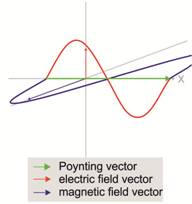Figure 1: Electromagnetic wave and right hand rule

The electric field of an electron consists of a static electric field. The electric field of an electromagnetic wave is a dynamic field. The electron carries a charge e. The electric field lines point to the center of the electron and is a spherically symmetrical field and do not move.

The electron also has a magnetic moment μs and also a magnetic field. What the magnetic field looks like is not known.

It is known, that electrons and positrons can be generated from an electromagnetic wave. We know the wavelength of the electromagnetic wave from the law of conservation of energy. The wavelength is the Compton wavelength of the electron (2.426 * 10-12 m) which is much larger than the radius from the particle model of an electron (10-13-10-15 m). This electromagnetic wave must be “captured” in the area in which the electron is created. (The antiparticle is ignored here and will be later examined.) For this “light trap” there are already considerations of other authors:

Herbert Weiß describes 1991 this “light trap”, in his book „Wellenmodell eines Teilchens“, as two mirrors. Between the mirrors the light jumps back and forth. Because the lepton forms a light clock in this model, it explains very clearly all the phenomena of the Lorenz transformation and the special theory of relativity.

Christoph Caesar describes the „model of the lepton as rotating photon or electromagnetic knot with internal twist” .

Carl-Friedrich Meyer describes the “light traps” in the same way as Christoph Caesar, with his electromagnetic elementary model for electrons, protons and neutrons.

In both electron models, the light rotates in a circle with an additional phase rotation of 180° at each rotation. Meyer explains very clearly why the magnetic field and the spin changes when the electron is rotated 360 degrees.

In this paper the lepton will be describe as a rotating wave, with internal twist. A cycle in an eight without internal twist is also possible and would bring the same results for the elementary charge.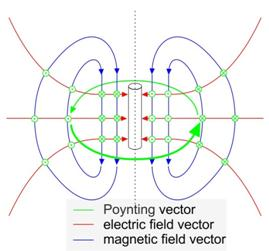Figure 2:&xnbsp; The electromagnetc field of an electron

There are three vector fields in an electromagnetic wave. All this three vectors must be at right angle to each other in each point of the space:

1.      The field lines of the Poynting vector point in the direction of energy transport. In this model the Poynting vector moves in a circular motion, with torsion by 180 ° at each half oscillation.

2.      The electric field: Due to the torsion by 180 degree with each half oscillation, the electric field lines always point to the center of the axis of rotation.

3.      The magnetic field lines are similar to the field of a bar magnet.

The starting point of electric field lines is always a positive charge. The endpoint is a negative charge. An electromagnetic wave has no charges and the electric field lines form a loop. For an electromagnetic wave that sits in the “light trap” and has become an electron, the electric field lines must be end. The electric field lines point to the axis of rotation of the Poynting vector and they are concentrated there.

The density of energy increases strongly with the concentration of the electric field lines at the axes of rotation. The density of energy becomes so strong that an event horizon forms on the axes of rotation. On the event horizon, the electric field lines will be end and form an elementary charge. It will be shown in the following text that the event horizon does not look like a ball. The event horizon looks like a string or a cylinder. The diameter is much smaller than the height of the cylinder. The cylinder is referred here as elementary cylinder.Figure 3: The electric field lines end on the event horizon

# 2.      Calculation the elementary charge e

The energy of the elementary particle is stored only in the electromagnetic field, because the particle consists only of light. The total energy Etotal of the elementary particle we describe with the Compton wavelength λ0, the speed of light c and the Planck's quantum h.( 2.1 )

The plan for calculating the elementary charge looks like this:

The total energy of the particle (function of λ0) is set equal to the energy of the electromagnetic field (function of e). Then we convert this equation to the charge e. The function for the elementary charge e will be dependent on λ0. After solving this problem, we see that the Compton wavelength λ0, has only a very little effect on the elementary charge e. That is what we expected.

## 2.1. The energy of the electromagnetic field

To calculate the energy of the electromagnetic field we take a close look at the field:

The electromagnetic field changes its shape with the distance to the center. From a long distance, it is the spherically symmetric field of a point charge. This field is called here sphere field. The electromagnetic wave can’t be concentrated to one point because of the Heisenberg uncertainty principle. Closer to the event horizon, it is the cylindrical field of a line charge. This field is called here cylindrical field.

For an easier calculation we only use these two field forms here. We have to find a boundary between the sphere field and the cylindrical field. In nature, there is no sharp boundary as in this calculation. There is a slow transition from the sphere field to the cylindrical field. This calculation is just a rough approximation. I choose the boundary as follows: The boundary between the sphere field and the cylindrical field is at the radius R, where the sphere surface is equal to the surface of the cylinder with the radius R and the height h. Another boundary between the two fields gives nearly the same results in the calculation.

For the boundary radius R we get the following equation: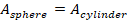( 2.2 )

hcylinder is the effective height of the cylinder. For the energy we know:( 2.3 )

We can compare the electric field vector from the cylindrical field with the field in a cylindrical capacitor. This equation is known:( 2.4 )

The magnetic and electric field energy are the same for an electromagnetic wave, because the energy alternates between electrical field energy and magnetic field energy. We can use only the electric energy density in the equation and simply double it, to consider the energy from the magnetic field.

For the energy density of the electric field we know:&xnbsp;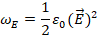For the energy density of the magnetic field we know:&xnbsp;For the energy density of the cylindrical field we get:The energy of the cylindrical field will be obtained by integrating the energy density over the cylinder volume from the boundary radius R to the event horizon rEH, this is the minimum possible radius.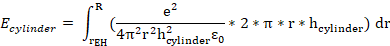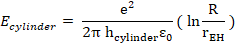( 2.5 )

In the same way we can calculate the energy of the sphere field.

The electric sphere field is equal to an electric field of a point charge: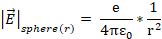For the energy density we get:&xnbsp;&xnbsp;&xnbsp;&xnbsp;&xnbsp;&xnbsp;&xnbsp;&xnbsp;&xnbsp;&xnbsp;&xnbsp;&xnbsp;&xnbsp;&xnbsp;&xnbsp;&xnbsp;&xnbsp;&xnbsp;&xnbsp;&xnbsp;&xnbsp; &xnbsp;&xnbsp;&xnbsp;&xnbsp;(2.6)

For the energy of the sphere field we must integrating the energy density over the sphere volume from the boundary radius R to infinity.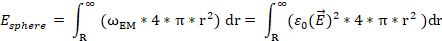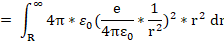( 2.7 )

We connect the equation (2.3) with the equation (2.7) and (2.5):( 2.3 )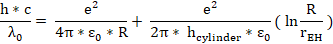( 2.9 )

## 2.2. The cylinder height: h cylinder

The height hcylinder of the elementary cylinder is unknown and not measured.

The height has a strong impact on the result and is thus the biggest weakness in this calculation.

With&xnbsp;&xnbsp;you get the correct result for the elementary charge. Here is an explanation:

For the determination of the cylinder height hcylinder we investigate the electromagnetic oscillation. We assume a sinusoidal oscillation.

The magnetic oscillation is recorded on a long paper as a wave. This paper is rolled up.Figure 4: The cylinder heightThe cylinder is as high as the magnetic oscillation in the positive and negative direction of the cylinder.

Fig. 2 shows that the magnetic field lines form a loop.&xnbsp; If this loop is λ0 long and is running in a circle, the maximum height is λ0 divided by Pi: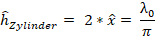( 2.10 )

The height of the elementary cylinder is proportional to the Compton wavelength

λ0 of the lepton. This means that the lepton with more energy will have a smaller elementary cylinder.

For the calculation with energy we need the RMS value of the height and have to divide the maximum value by the root of 2.( 2.11 )

The boundary R (2.2) between the sphere field and the cylindrical field can be used in the energy equation (2.9):We bring the elementary charge to the left side of the equation:With the equation for the cylinder height (2.11) we get:( 2.12 )

The last unknown is the radius for the event horizon rEH.

## 2.3. The event horizon

The event horizon is the limit curvature of space, where the time stand still or the speed of light will be zero for an outside observer. In this case, the electric field lines end at this limit. We have labeled the event horizon rEH in the upper equations. For a better understanding we will first calculate the Schwarzschild radius for a sphere mass m1 and then we calculate the event horizon for the elementary cylinder in the same way.

### 2.3.1. Schwarzschild radius for a sphere mass

An event horizon is similar to the beginning of a black hole. The event horizon starts where the light no longer manages to escape, because the space curvature is too high. For the calculation of the Schwarzschild radius we think of light as a particle with the mass m2, which tries to escape from the gravitational field of mass m1. The sphere mass m1 is in the center of coordinates. The light particle m2 is located on the event horizon at a distance rSchwarzschild from the origin of coordinates. The particle m2 moves away from the mass m1 with the speed of light v=c. We set the kinetic energy equal to the energy that the particle needs to escape from the gravitational field. The gravitational force F(r) is calculated by using the gravitational constant G of Newton. The energy is equal to power times way. Since the force changes with the distance r, we need the integral of the force F over the distance, from the starting point rSchwarzschild to infinity: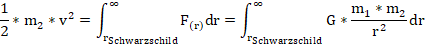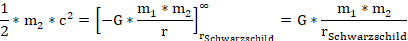### 2.3.2. The event horizon for the elementary cylinder

Figure 4 shows a model, how light is captured and how an elementary particle is created. The model is an electromagnetic wave rotating in a cylindrical way. Now the light tries to escape from its own gravitational field. The event horizon for the elementary particle is determined in the same way as the Schwarzschild radius of a point mass was determined. The same formula is used as for a point mass. This can be explained by the fact that the electromagnetic wave consists of a punctiform photon. This photon moves in the direction of the Poynting vector and oscillates with the amplitude λ0 through π in the direction of the magnetic field (blue line in Fig. 4). The punctiform photon is somewhere on the surface of the elementary cylinder. The energy of the electromagnetic wave is distributed with the electromagnetic field throughout the room.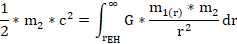The mass m1 is not a point mass, it is cylindrically. We can calculate the mass m1 from the energy inside an electromagnetic field as a function from the radius r.Equation (2.5) gives the energy in the cylinder from the event horizon to R. If we replace R by r, we have a function of the energy E (r) inside an imaginary cylinder of the radius r. For the mass m1 (r) the result is: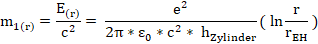If we insert the mass m1 (r) into the upper integral and cut out m2, we get this: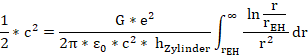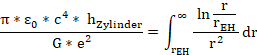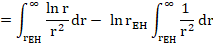For the calculation of the integral see appendix calculation B.Insert the cylinder height from equation (2.11) and it follows: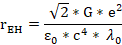(2.13)

This is the equation for the event horizon rEH of the elementary cylinder.

## 2.4. Summary and numerical solution for e

To determine the elementary charge e we have two equations (2.12) and (2.13) with two unknowns:( 2.13 )( 2.12 )

We know:

ε0 the electric field constant: 8.8541 * 10-12&xnbsp; (As)/(Vm)

h Planck’s constant of action: 6.626 * 10-34 Js

c the speed of light: 299 792 500 m/s

λ0 the Compton wavelength:

&xnbsp;&xnbsp;&xnbsp;&xnbsp;&xnbsp;&xnbsp;&xnbsp; for an electron: λ0 = 2.42631&xnbsp; * 10-12 m

&xnbsp;&xnbsp;&xnbsp;&xnbsp;&xnbsp;&xnbsp;&xnbsp; for an muon: λ0 = 1.17298 * 10-14 m

&xnbsp;&xnbsp;&xnbsp;&xnbsp;&xnbsp;&xnbsp;&xnbsp; for an tau: λ0 = 6.97716 * 10-16 m

G the gravitational constant: 6.674 * 10-11 (m3)/(kg *s2)

The elementary charge e can be calculated easy with these formulas in a table processing program. For the calculation we start for example with the event horizon radius by 10-20 m. After 4 steps we get a very good solution.

In the calculation the sign +- of the charge gets lost because of the e². By definition, the electron naturally carries a negative charge.

electron

 steps r EH m e calculated C e C 1 10-20 3.705*10-19 2 7.465*10-59 1.53248*10-19 3 1.277*10-59 1.51986*10-19 1.602*10-19 4 1.256*10-59 1.51975*10-19 94.86 %

muon

 steps r EH m e calculated C e C 1 10-20 4.410*10-19 2 2.188*10-56 1.619*10-19 3 2.949*10-57 1.602*10-19 1.602*10-19 4 2.888*10-57 1.602*10-19 99.997 %

tau

 steps r EH m e calculated C e C 1 10-20 4.995*10-19 2 4.7196*10-55 1.672*10-19 3 5.2858*10-56 1.652*10-19 1.602*10-19 4 5.1589*10-56 1.651*10-19 103.08 %

# 1.      Conclusion and outlook

The field lines can reach to the event horizon and end or start there. This is not possible with the model of a sphere charge, because in that case the charge would be much too small. The formation of charges by the model of an electromagnetic elementary field can be explained.&xnbsp; The energy of the particle has little influence on the elementary charge e. The tau is 3477 times heavy as the electron, but the calculated elementary charge is only 1.086 higher. However, the energy is not quite out as expected. This means, that this calculation is not quite correct or we have an excess of positive charge (the proton is heavier than the electron).

The errors are smaller than expected. Many approximations were used:

The inaccuracy of this calculation is due to the simplified transition from the cylinder field to the sphere field and we use only the cylinder field to calculate the event horizon radius. In this calculation, we did not include the energy behind the event horizon radius, the energy inside the elementary cylinder. The field lines end abruptly at the event horizon and do not fall slowly into the gravitational funnel.

2/3 elementary charges or 1/3 elementary charges, as we know from the quarks, can’t be calculated with these equations.

The dimensions of the event horizon (elementary cylinder) are very important for other considerations. The event horizon is much higher than the cylinder’s diameter (factor 1040-1047). The elementary cylinder looks like a string from the string theory but is not one – dimensional. That’s important for the gravity.

With this model, quantum gravity can be easily understood and calculated.

The cylinder’s diameter is inversely proportional to the Compton wavelength. The diameter is proportional to the energy of the elementary particle.

An electromagnetic wave that moves to a mass, gets more energy (blue shift). The diameter becomes larger with a stronger gravitational field. The elementary cylinder in the gravitational field becomes a truncated cone. If we consider the elementary particle as a light clock, the time will be change by gravitation. This can be calculated very clearly. The gravitational acceleration can also be calculated with the course of the Poynting vector on the truncated cone. The gravitational force can be calculated from the course of the Poynting vector and the radiation pressure of the Poynting vector. The fine structure constant α is the ratio of the energy behind the event horizon radius (energy density at the event horizon multiplied by the volume of the elementary cylinder and root (2)) to the total energy of the particle.

# 2.      Appendix

## 4.1. Calculation B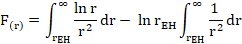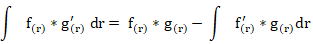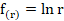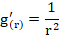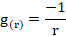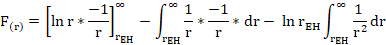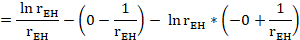## 4.3.General formulas

Mass - energy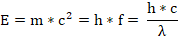Speed of light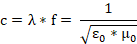Fine structure constant: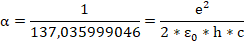Kinetic energy: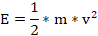Electric field of a cylinder in vacuum: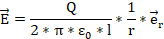Electric field of a sphere charge in vacuum: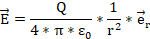Energy density of electromagnetic fields in vacuum: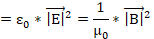# 3.      References

J. Brandes /J. Czerniawski; Spezielle und Allgemeine Relativitätstheorie für Physiker und Philosophen;

Karlsbad: VRI - Verlag relativistischer Interpretationen; 2010

ISBN 978-3-930879-08-3

Caesar, Christoph; www.ccaesar.com/ger_index.html, 2003-2019

Patentschrift DE10341341 A1 (Offenlegungsschrift), eingereicht am 8.9.2003, offengelegt am 14.4.2005

R. P. Feynman; QED Die seltsame Theorie des Lichts und der Materie;

München: R. Piper GmbH & Co. KG; 1995; ISBN 3-492-11562-4

Feynman/Leighton/Sands; Feynman - Vorlesungen über Physik (Band 1 bis3);

München: R. Oldenbourg Verlag; 1987; ISBN 3-486-20018-6

Feynman; Quantenelektrodynamik (Band 3a);

München: R. Oldenbourg Verlag; 1992; ISBN 3-486-22315-1

Fließbach, T.; Allgemeine Relativitätstheorie;

Heidelberg: Springer - Spektrum Akademischer Verlag; 2012

ISBN 978-3-8274-3031-1

Frohne, H.; Einführung in die Elektrotechnik (1-3)

Grundlagen und Netzwerke,

Elektrische und magnetische Felder,

Wechselstrom,

Teubner Studienskripten; Stuttgart, 1982, 1989, 1989

Gößling, Manuel; Physik – Rechnen mit dem Elementarzylinder

Das Elektron als elektromagnetische Welle

2. Auflage 2018; ISBN 978-3-9819366-1-2

Meyer, Carl-Friedrich; Relativistische invariante Bahnen in Elementarteilchen,

Shaker Verlag, Aachen 2005; ISBN 3-8322-3692-9

Weiß, H.; Wellenmodell eines Teilchens;

Unterhaching: Herbert Weiß; 1991

http://www.slac.stanford.edu/exp/e144/e144.html; 9-2019

Wellenmodell eines Teilchens; Unterhaching: Herbert Weiß; 1991

&xnbsp; Christoph Caesar, www.ccaesar.com/ger_index.html, 2003-2019

Carl-Friedrich Meyer, Relativistische invariante Bahnen in Elementarteilchen, Aachen 2005

 This is twice the energy of the electric field.

https://de.wikipedia.org/wiki/Elektrisches_Feld#Weitere_Beispiele_für

_elektrische_Felder

 This event horizon can’t capture photons. The wavelength of the photons is much larger than the diameter of the event horizon. The reason of the circular motion of the photon is the charge in the magnetic field and not to the curvature of the space.